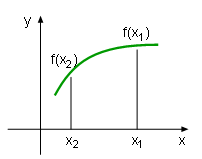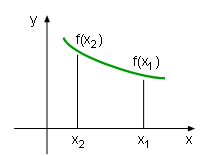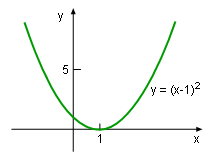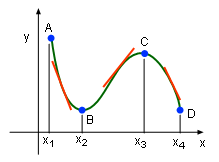Ch 4. The Mean Value Theorem Multimedia Engineering Math Maximum & Minimum Rolle'sTheorem Mean ValueTheorem MonotonicFunctions First DerivativeTest Concavity &Inflection SecondDerivative Test
 Chapter 1. Limits 2. Derivatives I 3. Derivatives II 4. Mean Value 5. Curve Sketching 6. Integrals 7. Inverse Functions 8. Integration Tech. 9. Integrate App. 10. Parametric Eqs. 11. Polar Coord. 12. Series Appendix Basic Math Units Search eBooks Dynamics Fluids Math Mechanics Statics Thermodynamics Author(s): Hengzhong Wen Chean Chin Ngo Meirong Huang Kurt Gramoll ©Kurt GramollMATHEMATICS - THEORY In this section, the concept of a monotonic function is discussed and the method to find a function's monotonicity is introduced. MonotonicityIncreasing FunctionDecreasing Function In engineering research, sometimes a diagram can help the researcher better understand a function. A function's increasing or decreasing tendency is useful when sketching a draft. A function is called increasing on an interval if the function value increases as the independent value increases. That is if x1 > x2, then f(x1) > f(x2). On the other hand, a function is called decreasing on an interval if the function value decreases as the independent value increases. That is if x1 > x2, then f(x1) < f(x2). A function's increasing or decreasing tendency is called monotonicity on its domain.Example of Monotonic Function The monotonicity concept can be better understood by finding the increasing and decreasing interval of the function, say y = (x-1)2. In the interval of (-∞, 1], the function is decreasing. In the interval of [1, +∞), the function is increasing. However, the function is not monotonic in its domain (-∞, +∞).Derivative and Monotonic In the Derivative and Monotonic graphic on the left, the function is decreasing in [x1, x2] and [x3, x4], and the slope of the function's tangent lines are negative. On the other hand, the function is increasing in [x2, x3] and the slope of the function's tangent line is positive. Is there any certain relationship between monotonicity and derivative? The answer is yes and is discussed below. Test for Monotonic Functions Test for monotonic functions states: Suppose a function is continuous on [a, b] and it is differentiable on (a, b). If the derivative is larger than zero for all x in (a, b), then the function is increasing on [a, b]. If the derivative is less than zero for all x in (a, b), then the function is decreasing on [a, b].Function y = x2 - 4 The test for monotonic functions can be better understood by finding the increasing and decreasing range for the function f(x) = x2 - 4. The function f(x) = x2 - 4 is a polynomial function, it is continuous and differentiable in its domain (-∞, +∞), and thus it satisfies the condition of monatomic function test. In order to find its monotonicity, the derivative of the function needs to be calculated. That is      df(x)/dx = d(x2 - 4)/dx                   = (d(x2) - d(4))/dx                      = 2x It is obvious that the function df(x)/dx = 2x is negative when x < 0, and it is positive when x > 0. Therefore, function f(x) = x2 - 4 is increasing in the range of (-∞, 0) and decreasing in the range of (0, +∞). This result is confirmed by the diagram on the left.